# 1. Orthogonal Projections and Their Applications¶

## 1.1. Overview¶

Orthogonal projection is a cornerstone of vector space methods, with many diverse applications.

These include

• Least squares projection, also known as linear regression

• Conditional expectations for multivariate normal (Gaussian) distributions

• Gram–Schmidt orthogonalization

• QR decomposition

• Orthogonal polynomials

• etc

In this lecture, we focus on

• key ideas

• least squares regression

We’ll require the following imports:

import numpy as np
from scipy.linalg import qr


For background and foundational concepts, see our lecture on linear algebra.

For more proofs and greater theoretical detail, see A Primer in Econometric Theory.

For a complete set of proofs in a general setting, see, for example, [Rom05].

For an advanced treatment of projection in the context of least squares prediction, see this book chapter.

## 1.2. Key Definitions¶

Assume $$x, z \in \mathbb R^n$$.

Define $$\langle x, z\rangle = \sum_i x_i z_i$$.

Recall $$\|x \|^2 = \langle x, x \rangle$$.

The law of cosines states that $$\langle x, z \rangle = \| x \| \| z \| \cos(\theta)$$ where $$\theta$$ is the angle between the vectors $$x$$ and $$z$$.

When $$\langle x, z\rangle = 0$$, then $$\cos(\theta) = 0$$ and $$x$$ and $$z$$ are said to be orthogonal and we write $$x \perp z$$.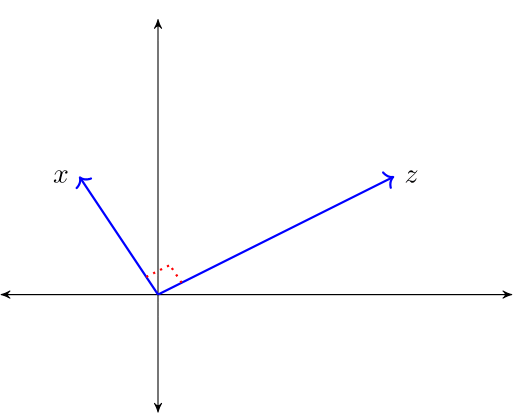For a linear subspace $$S \subset \mathbb R^n$$, we call $$x \in \mathbb R^n$$ orthogonal to $$S$$ if $$x \perp z$$ for all $$z \in S$$, and write $$x \perp S$$.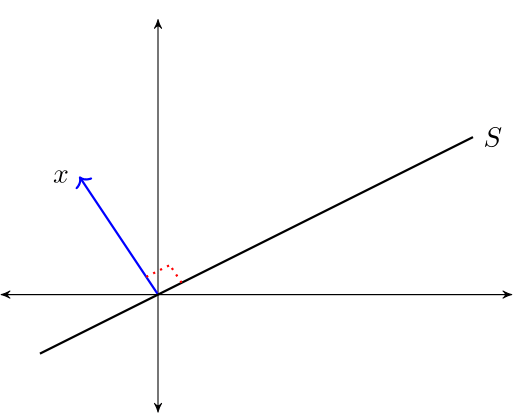The orthogonal complement of linear subspace $$S \subset \mathbb R^n$$ is the set $$S^{\perp} := \{x \in \mathbb R^n \,:\, x \perp S\}$$.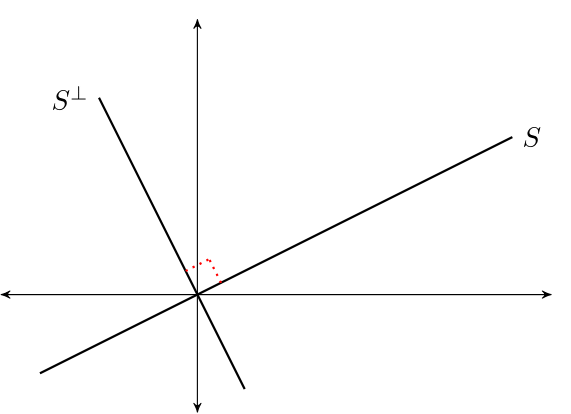$$S^\perp$$ is a linear subspace of $$\mathbb R^n$$

• To see this, fix $$x, y \in S^{\perp}$$ and $$\alpha, \beta \in \mathbb R$$.

• Observe that if $$z \in S$$, then

$\langle \alpha x + \beta y, z \rangle = \alpha \langle x, z \rangle + \beta \langle y, z \rangle = \alpha \times 0 + \beta \times 0 = 0$
• Hence $$\alpha x + \beta y \in S^{\perp}$$, as was to be shown

A set of vectors $$\{x_1, \ldots, x_k\} \subset \mathbb R^n$$ is called an orthogonal set if $$x_i \perp x_j$$ whenever $$i \not= j$$.

If $$\{x_1, \ldots, x_k\}$$ is an orthogonal set, then the Pythagorean Law states that

$\| x_1 + \cdots + x_k \|^2 = \| x_1 \|^2 + \cdots + \| x_k \|^2$

For example, when $$k=2$$, $$x_1 \perp x_2$$ implies

$\| x_1 + x_2 \|^2 = \langle x_1 + x_2, x_1 + x_2 \rangle = \langle x_1, x_1 \rangle + 2 \langle x_2, x_1 \rangle + \langle x_2, x_2 \rangle = \| x_1 \|^2 + \| x_2 \|^2$

### 1.2.1. Linear Independence vs Orthogonality¶

If $$X \subset \mathbb R^n$$ is an orthogonal set and $$0 \notin X$$, then $$X$$ is linearly independent.

Proving this is a nice exercise.

While the converse is not true, a kind of partial converse holds, as we’ll see below.

## 1.3. The Orthogonal Projection Theorem¶

What vector within a linear subspace of $$\mathbb R^n$$ best approximates a given vector in $$\mathbb R^n$$?

The next theorem answers this question.

Theorem (OPT) Given $$y \in \mathbb R^n$$ and linear subspace $$S \subset \mathbb R^n$$, there exists a unique solution to the minimization problem

$\hat y := \argmin_{z \in S} \|y - z\|$

The minimizer $$\hat y$$ is the unique vector in $$\mathbb R^n$$ that satisfies

• $$\hat y \in S$$

• $$y - \hat y \perp S$$

The vector $$\hat y$$ is called the orthogonal projection of $$y$$ onto $$S$$.

The next figure provides some intuition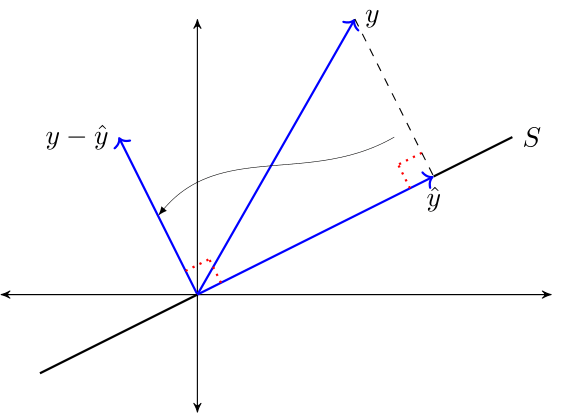### 1.3.1. Proof of Sufficiency¶

We’ll omit the full proof.

But we will prove sufficiency of the asserted conditions.

To this end, let $$y \in \mathbb R^n$$ and let $$S$$ be a linear subspace of $$\mathbb R^n$$.

Let $$\hat y$$ be a vector in $$\mathbb R^n$$ such that $$\hat y \in S$$ and $$y - \hat y \perp S$$.

Let $$z$$ be any other point in $$S$$ and use the fact that $$S$$ is a linear subspace to deduce

$\| y - z \|^2 = \| (y - \hat y) + (\hat y - z) \|^2 = \| y - \hat y \|^2 + \| \hat y - z \|^2$

Hence $$\| y - z \| \geq \| y - \hat y \|$$, which completes the proof.

### 1.3.2. Orthogonal Projection as a Mapping¶

For a linear space $$Y$$ and a fixed linear subspace $$S$$, we have a functional relationship

$y \in Y\; \mapsto \text{ its orthogonal projection } \hat y \in S$

By the OPT, this is a well-defined mapping or operator from $$\mathbb R^n$$ to $$\mathbb R^n$$.

In what follows we denote this operator by a matrix $$P$$

• $$P y$$ represents the projection $$\hat y$$.

• This is sometimes expressed as $$\hat E_S y = P y$$, where $$\hat E$$ denotes a wide-sense expectations operator and the subscript $$S$$ indicates that we are projecting $$y$$ onto the linear subspace $$S$$.

The operator $$P$$ is called the orthogonal projection mapping onto $$S$$.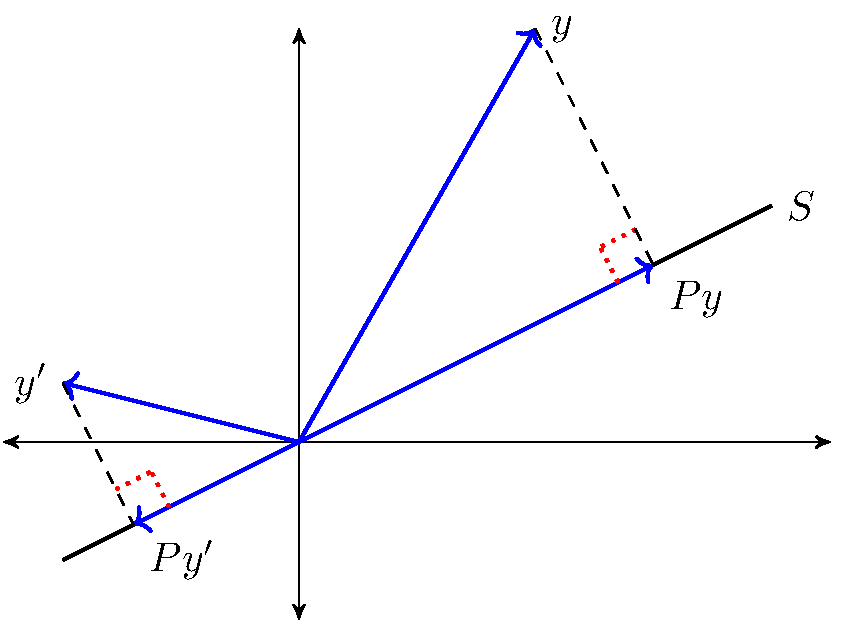It is immediate from the OPT that for any $$y \in \mathbb R^n$$

1. $$P y \in S$$ and

2. $$y - P y \perp S$$

From this, we can deduce additional useful properties, such as

1. $$\| y \|^2 = \| P y \|^2 + \| y - P y \|^2$$ and

2. $$\| P y \| \leq \| y \|$$

For example, to prove 1, observe that $$y = P y + y - P y$$ and apply the Pythagorean law.

#### 1.3.2.1. Orthogonal Complement¶

Let $$S \subset \mathbb R^n$$.

The orthogonal complement of $$S$$ is the linear subspace $$S^{\perp}$$ that satisfies $$x_1 \perp x_2$$ for every $$x_1 \in S$$ and $$x_2 \in S^{\perp}$$.

Let $$Y$$ be a linear space with linear subspace $$S$$ and its orthogonal complement $$S^{\perp}$$.

We write

$Y = S \oplus S^{\perp}$

to indicate that for every $$y \in Y$$ there is unique $$x_1 \in S$$ and a unique $$x_2 \in S^{\perp}$$ such that $$y = x_1 + x_2$$.

Moreover, $$x_1 = \hat E_S y$$ and $$x_2 = y - \hat E_S y$$.

This amounts to another version of the OPT:

Theorem. If $$S$$ is a linear subspace of $$\mathbb R^n$$, $$\hat E_S y = P y$$ and $$\hat E_{S^{\perp}} y = M y$$, then

$P y \perp M y \quad \text{and} \quad y = P y + M y \quad \text{for all } \, y \in \mathbb R^n$

The next figure illustrates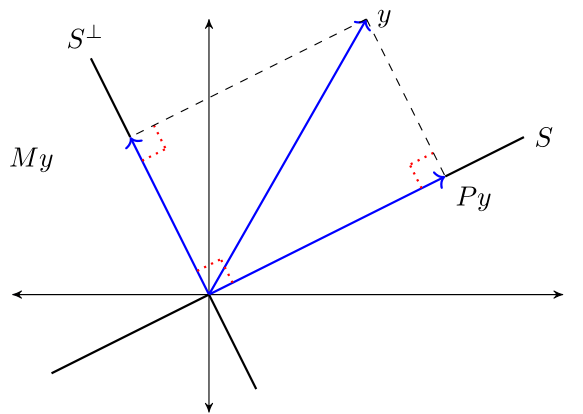## 1.4. Orthonormal Basis¶

An orthogonal set of vectors $$O \subset \mathbb R^n$$ is called an orthonormal set if $$\| u \| = 1$$ for all $$u \in O$$.

Let $$S$$ be a linear subspace of $$\mathbb R^n$$ and let $$O \subset S$$.

If $$O$$ is orthonormal and $$\mathop{\mathrm{span}} O = S$$, then $$O$$ is called an orthonormal basis of $$S$$.

$$O$$ is necessarily a basis of $$S$$ (being independent by orthogonality and the fact that no element is the zero vector).

One example of an orthonormal set is the canonical basis $$\{e_1, \ldots, e_n\}$$ that forms an orthonormal basis of $$\mathbb R^n$$, where $$e_i$$ is the $$i$$ th unit vector.

If $$\{u_1, \ldots, u_k\}$$ is an orthonormal basis of linear subspace $$S$$, then

$x = \sum_{i=1}^k \langle x, u_i \rangle u_i \quad \text{for all} \quad x \in S$

To see this, observe that since $$x \in \mathop{\mathrm{span}}\{u_1, \ldots, u_k\}$$, we can find scalars $$\alpha_1, \ldots, \alpha_k$$ that verify

(1.1)$x = \sum_{j=1}^k \alpha_j u_j$

Taking the inner product with respect to $$u_i$$ gives

$\langle x, u_i \rangle = \sum_{j=1}^k \alpha_j \langle u_j, u_i \rangle = \alpha_i$

Combining this result with (1.1) verifies the claim.

### 1.4.1. Projection onto an Orthonormal Basis¶

When a subspace onto which we project is orthonormal, computing the projection simplifies:

Theorem If $$\{u_1, \ldots, u_k\}$$ is an orthonormal basis for $$S$$, then

(1.2)$P y = \sum_{i=1}^k \langle y, u_i \rangle u_i, \quad \forall \; y \in \mathbb R^n$

Proof: Fix $$y \in \mathbb R^n$$ and let $$P y$$ be defined as in (1.2).

Clearly, $$P y \in S$$.

We claim that $$y - P y \perp S$$ also holds.

It sufficies to show that $$y - P y \perp$$ any basis vector $$u_i$$.

This is true because

$\left\langle y - \sum_{i=1}^k \langle y, u_i \rangle u_i, u_j \right\rangle = \langle y, u_j \rangle - \sum_{i=1}^k \langle y, u_i \rangle \langle u_i, u_j \rangle = 0$

(Why is this sufficient to establish the claim that $$y - P y \perp S$$?)

## 1.5. Projection Via Matrix Algebra¶

Let $$S$$ be a linear subspace of $$\mathbb R^n$$ and let $$y \in \mathbb R^n$$.

We want to compute the matrix $$P$$ that verifies

$\hat E_S y = P y$

Evidently $$Py$$ is a linear function from $$y \in \mathbb R^n$$ to $$P y \in \mathbb R^n$$.

This reference is useful.

Theorem. Let the columns of $$n \times k$$ matrix $$X$$ form a basis of $$S$$. Then

$P = X (X'X)^{-1} X'$

Proof: Given arbitrary $$y \in \mathbb R^n$$ and $$P = X (X'X)^{-1} X'$$, our claim is that

1. $$P y \in S$$, and

2. $$y - P y \perp S$$

Claim 1 is true because

$P y = X (X' X)^{-1} X' y = X a \quad \text{when} \quad a := (X' X)^{-1} X' y$

An expression of the form $$X a$$ is precisely a linear combination of the columns of $$X$$ and hence an element of $$S$$.

Claim 2 is equivalent to the statement

$y - X (X' X)^{-1} X' y \, \perp\, X b \quad \text{for all} \quad b \in \mathbb R^K$

To verify this, notice that if $$b \in \mathbb R^K$$, then

$(X b)' [y - X (X' X)^{-1} X' y] = b' [X' y - X' y] = 0$

The proof is now complete.

### 1.5.1. Starting with the Basis¶

It is common in applications to start with $$n \times k$$ matrix $$X$$ with linearly independent columns and let

$S := \mathop{\mathrm{span}} X := \mathop{\mathrm{span}} \{\col_1 X, \ldots, \col_k X \}$

Then the columns of $$X$$ form a basis of $$S$$.

From the preceding theorem, $$P = X (X' X)^{-1} X' y$$ projects $$y$$ onto $$S$$.

In this context, $$P$$ is often called the projection matrix

• The matrix $$M = I - P$$ satisfies $$M y = \hat E_{S^{\perp}} y$$ and is sometimes called the annihilator matrix.

### 1.5.2. The Orthonormal Case¶

Suppose that $$U$$ is $$n \times k$$ with orthonormal columns.

Let $$u_i := \mathop{\mathrm{col}} U_i$$ for each $$i$$, let $$S := \mathop{\mathrm{span}} U$$ and let $$y \in \mathbb R^n$$.

We know that the projection of $$y$$ onto $$S$$ is

$P y = U (U' U)^{-1} U' y$

Since $$U$$ has orthonormal columns, we have $$U' U = I$$.

Hence

$P y = U U' y = \sum_{i=1}^k \langle u_i, y \rangle u_i$

We have recovered our earlier result about projecting onto the span of an orthonormal basis.

### 1.5.3. Application: Overdetermined Systems of Equations¶

Let $$y \in \mathbb R^n$$ and let $$X$$ be $$n \times k$$ with linearly independent columns.

Given $$X$$ and $$y$$, we seek $$b \in \mathbb R^k$$ that satisfies the system of linear equations $$X b = y$$.

If $$n > k$$ (more equations than unknowns), then $$b$$ is said to be overdetermined.

Intuitively, we may not be able to find a $$b$$ that satisfies all $$n$$ equations.

The best approach here is to

• Accept that an exact solution may not exist.

• Look instead for an approximate solution.

By approximate solution, we mean a $$b \in \mathbb R^k$$ such that $$X b$$ is close to $$y$$.

The next theorem shows that a best approximation is well defined and unique.

The proof uses the OPT.

Theorem The unique minimizer of $$\| y - X b \|$$ over $$b \in \mathbb R^K$$ is

$\hat \beta := (X' X)^{-1} X' y$

Proof: Note that

$X \hat \beta = X (X' X)^{-1} X' y = P y$

Since $$P y$$ is the orthogonal projection onto $$\mathop{\mathrm{span}}(X)$$ we have

$\| y - P y \| \leq \| y - z \| \text{ for any } z \in \mathop{\mathrm{span}}(X)$

Because $$Xb \in \mathop{\mathrm{span}}(X)$$

$\| y - X \hat \beta \| \leq \| y - X b \| \text{ for any } b \in \mathbb R^K$

This is what we aimed to show.

## 1.6. Least Squares Regression¶

Let’s apply the theory of orthogonal projection to least squares regression.

This approach provides insights about many geometric properties of linear regression.

We treat only some examples.

### 1.6.1. Squared Risk Measures¶

Given pairs $$(x, y) \in \mathbb R^K \times \mathbb R$$, consider choosing $$f \colon \mathbb R^K \to \mathbb R$$ to minimize the risk

$R(f) := \mathbb{E}\, [(y - f(x))^2]$

If probabilities and hence $$\mathbb{E}\,$$ are unknown, we cannot solve this problem directly.

However, if a sample is available, we can estimate the risk with the empirical risk:

$\min_{f \in \mathcal{F}} \frac{1}{N} \sum_{n=1}^N (y_n - f(x_n))^2$

Minimizing this expression is called empirical risk minimization.

The set $$\mathcal{F}$$ is sometimes called the hypothesis space.

The theory of statistical learning tells us that to prevent overfitting we should take the set $$\mathcal{F}$$ to be relatively simple.

If we let $$\mathcal{F}$$ be the class of linear functions, the problem is

$\min_{b \in \mathbb R^K} \; \sum_{n=1}^N (y_n - b' x_n)^2$

This is the sample linear least squares problem.

### 1.6.2. Solution¶

Define the matrices

$\begin{split} y := \left( \begin{array}{c} y_1 \\ y_2 \\ \vdots \\ y_N \end{array} \right), \quad x_n := \left( \begin{array}{c} x_{n1} \\ x_{n2} \\ \vdots \\ x_{nK} \end{array} \right) = n\text{-th obs on all regressors} \end{split}$

and

$\begin{split} X := \left( \begin{array}{c} x_1' \\ x_2' \\ \vdots \\ x_N' \end{array} \right) :=: \left( \begin{array}{cccc} x_{11} & x_{12} & \cdots & x_{1K} \\ x_{21} & x_{22} & \cdots & x_{2K} \\ \vdots & \vdots & & \vdots \\ x_{N1} & x_{N2} & \cdots & x_{NK} \end{array} \right) \end{split}$

We assume throughout that $$N > K$$ and $$X$$ is full column rank.

If you work through the algebra, you will be able to verify that $$\| y - X b \|^2 = \sum_{n=1}^N (y_n - b' x_n)^2$$.

Since monotone transforms don’t affect minimizers, we have

$\argmin_{b \in \mathbb R^K} \sum_{n=1}^N (y_n - b' x_n)^2 = \argmin_{b \in \mathbb R^K} \| y - X b \|$

By our results about overdetermined linear systems of equations, the solution is

$\hat \beta := (X' X)^{-1} X' y$

Let $$P$$ and $$M$$ be the projection and annihilator associated with $$X$$:

$P := X (X' X)^{-1} X' \quad \text{and} \quad M := I - P$

The vector of fitted values is

$\hat y := X \hat \beta = P y$

The vector of residuals is

$\hat u := y - \hat y = y - P y = M y$

Here are some more standard definitions:

• The total sum of squares is $$:= \| y \|^2$$.

• The sum of squared residuals is $$:= \| \hat u \|^2$$.

• The explained sum of squares is $$:= \| \hat y \|^2$$.

TSS = ESS + SSR

We can prove this easily using the OPT.

From the OPT we have $$y = \hat y + \hat u$$ and $$\hat u \perp \hat y$$.

Applying the Pythagorean law completes the proof.

## 1.7. Orthogonalization and Decomposition¶

Let’s return to the connection between linear independence and orthogonality touched on above.

A result of much interest is a famous algorithm for constructing orthonormal sets from linearly independent sets.

The next section gives details.

### 1.7.1. Gram-Schmidt Orthogonalization¶

Theorem For each linearly independent set $$\{x_1, \ldots, x_k\} \subset \mathbb R^n$$, there exists an orthonormal set $$\{u_1, \ldots, u_k\}$$ with

$\mathop{\mathrm{span}} \{x_1, \ldots, x_i\} = \mathop{\mathrm{span}} \{u_1, \ldots, u_i\} \quad \text{for} \quad i = 1, \ldots, k$

The Gram-Schmidt orthogonalization procedure constructs an orthogonal set $$\{ u_1, u_2, \ldots, u_n\}$$.

One description of this procedure is as follows:

• For $$i = 1, \ldots, k$$, form $$S_i := \mathop{\mathrm{span}}\{x_1, \ldots, x_i\}$$ and $$S_i^{\perp}$$

• Set $$v_1 = x_1$$

• For $$i \geq 2$$ set $$v_i := \hat E_{S_{i-1}^{\perp}} x_i$$ and $$u_i := v_i / \| v_i \|$$

The sequence $$u_1, \ldots, u_k$$ has the stated properties.

A Gram-Schmidt orthogonalization construction is a key idea behind the Kalman filter described in A First Look at the Kalman filter.

In some exercises below, you are asked to implement this algorithm and test it using projection.

### 1.7.2. QR Decomposition¶

The following result uses the preceding algorithm to produce a useful decomposition.

Theorem If $$X$$ is $$n \times k$$ with linearly independent columns, then there exists a factorization $$X = Q R$$ where

• $$R$$ is $$k \times k$$, upper triangular, and nonsingular

• $$Q$$ is $$n \times k$$ with orthonormal columns

Proof sketch: Let

• $$x_j := \col_j (X)$$

• $$\{u_1, \ldots, u_k\}$$ be orthonormal with the same span as $$\{x_1, \ldots, x_k\}$$ (to be constructed using Gram–Schmidt)

• $$Q$$ be formed from cols $$u_i$$

Since $$x_j \in \mathop{\mathrm{span}}\{u_1, \ldots, u_j\}$$, we have

$x_j = \sum_{i=1}^j \langle u_i, x_j \rangle u_i \quad \text{for } j = 1, \ldots, k$

Some rearranging gives $$X = Q R$$.

### 1.7.3. Linear Regression via QR Decomposition¶

For matrices $$X$$ and $$y$$ that overdetermine $$\beta$$ in the linear equation system $$y = X \beta$$, we found the least squares approximator $$\hat \beta = (X' X)^{-1} X' y$$.

Using the QR decomposition $$X = Q R$$ gives

\begin{split} \begin{aligned} \hat \beta & = (R'Q' Q R)^{-1} R' Q' y \\ & = (R' R)^{-1} R' Q' y \\ & = R^{-1} (R')^{-1} R' Q' y = R^{-1} Q' y \end{aligned} \end{split}

Numerical routines would in this case use the alternative form $$R \hat \beta = Q' y$$ and back substitution.

## 1.8. Exercises¶

Exercise 1.1

Show that, for any linear subspace $$S \subset \mathbb R^n$$, $$S \cap S^{\perp} = \{0\}$$.

Exercise 1.2

Let $$P = X (X' X)^{-1} X'$$ and let $$M = I - P$$. Show that $$P$$ and $$M$$ are both idempotent and symmetric. Can you give any intuition as to why they should be idempotent?

Exercise 1.3

Using Gram-Schmidt orthogonalization, produce a linear projection of $$y$$ onto the column space of $$X$$ and verify this using the projection matrix $$P := X (X' X)^{-1} X'$$ and also using QR decomposition, where:

$\begin{split} y := \left( \begin{array}{c} 1 \\ 3 \\ -3 \end{array} \right), \quad \end{split}$

and

$\begin{split} X := \left( \begin{array}{cc} 1 & 0 \\ 0 & -6 \\ 2 & 2 \end{array} \right) \end{split}$